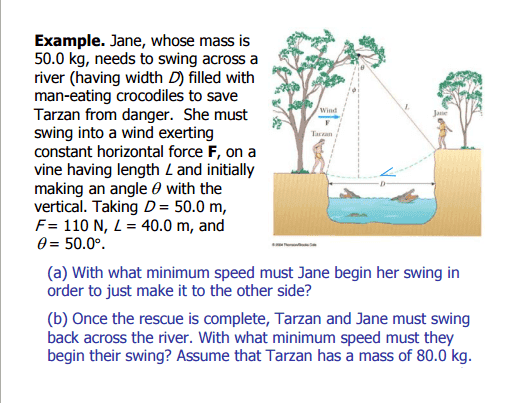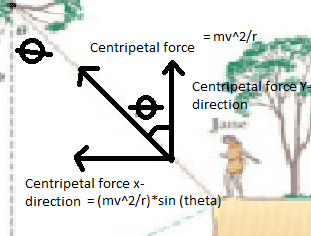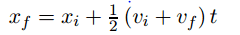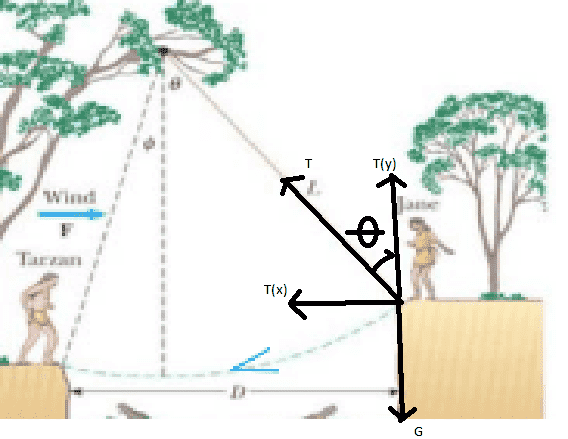# Possible to use centripetal acceleration for this problem?

## Homework Statement[/B]

Everywhere I looked they solved this problem with energy conservation, but is it possible to solve it using centripetal acceleration?

## Homework Equations## The Attempt at a Solution

I tried putting (mv^2/r)*sin (theta) - F= ma which i solved for a. This gives me acceleration in x axis?

Then I used kinematic equation:Did I think right, is it possible to use centripetal acceleration to solve this problem? Why? Why not? I got 8.6m/s as the initial speed I believe, the right answer is 6.15m/s

Chestermiller
Mentor
It's possible to solve this if you use force balances. But, to start with, you need to set up the force balance correctly. Do you know how to work with cylindrical coordinates? The centripetal force determines only the tension in the vine (radial direction). The important component of the force balance for purposes of this problem is in the tangential direction (which does not involve the centripetal acceleration).

Chet

It's possible to solve this if you use force balances. But, to start with, you need to set up the force balance correctly. Do you know how to work with cylindrical coordinates? The centripetal force determines only the tension in the vine (radial direction). The important component of the force balance for purposes of this problem is in the tangential direction (which does not involve the centripetal acceleration).

Chet

But could you please explain why I cant just decompose the radial acceleration in the x-axis. Is it because centripetal force is not a real force?

Chestermiller
Mentor
But could you please explain why I cant just decompose the radial acceleration in the x-axis. Is it because centripetal force is not a real force?
Partly. It's mainly because you did the force balance incorrectly. Let's see your free body diagram, and please call the tension that the vine exerts on the people T. Let x be the distance measured to the right from the point of attachment of the vine to the tree. Let y be the distance measured downward from the point of attachment of the vine to the tree. Let's see your force balance equations in the x and y directions.

Chet

Partly. It's mainly because you did the force balance incorrectly. Let's see your free body diagram, and please call the tension that the vine exerts on the people T. Let x be the distance measured to the right from the point of attachment of the vine to the tree. Let y be the distance measured downward from the point of attachment of the vine to the tree. Let's see your force balance equations in the x and y directions.

Chetma(y)=T(y)-G
ma(x)=T(x)-F

T=√(T(y)^2+T(x)^2)

mv^2/r=√((T(y)-G)^2+(T(x)-F)^2)) - is this one right?

Chestermiller
Mentor
View attachment 91963

ma(y)=T(y)-G
ma(x)=T(x)-F

T=√(T(y)^2+T(x)^2)

mv^2/r=√((T(y)-G)^2+(T(x)-F)^2)) - is this one right?
Your original force balances are OK, but not this final equation. It should read:
$$m\frac{v^2}{r}=T-Fsinθ-Gcosθ$$

•Warlic
Your original force balances are OK, but not this final equation. It should read:
$$m\frac{v^2}{r}=T-Fsinθ-Gcosθ$$
Thank you very much, could I just ask you one last question - how did you get that equation? It makes sense, when the vine is straight down its gonna be T-F*0-G*1= T-G
and if the vine is totally horizontal its gonna be T-F*1-G*0= T-F. But how did you see that it was gonna be -F*sin(theta) and - G*cos(theta). I dont see the correlation between theta and the forces F and G.

Chestermiller
Mentor
Thank you very much, could I just ask you one last question - how did you get that equation? It makes sense, when the vine is straight down its gonna be T-F*0-G*1= T-G
and if the vine is totally horizontal its gonna be T-F*1-G*0= T-F. But how did you see that it was gonna be -F*sin(theta) and - G*cos(theta). I dont see the correlation between theta and the forces F and G.
Fsin(theta) is the component of F along the vine and G cos(theta) is the component of G along the vine.
The thing that was wrong with your equation in post #5 is that it assumes that the acceleration is totally centripetal, and it is not. There is a component of acceleration in the direction tangent to the arc. The tangential velocity is changing along the arc. The equation that I wrote gives the component of the force balance in the direction perpendicular to the arc (along the vine).

Fsin(theta) is the component of F along the vine and G cos(theta) is the component of G along the vine.
The thing that was wrong with your equation in post #5 is that it assumes that the acceleration is totally centripetal, and it is not. There is a component of acceleration in the direction tangent to the arc. The tangential velocity is changing along the arc. The equation that I wrote gives the component of the force balance in the direction perpendicular to the arc (along the vine).
Fantastic! thank you :)

haruspex
•